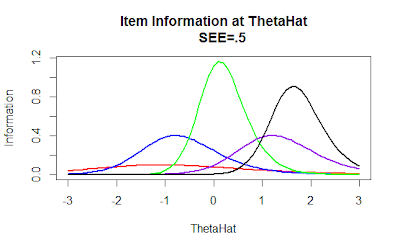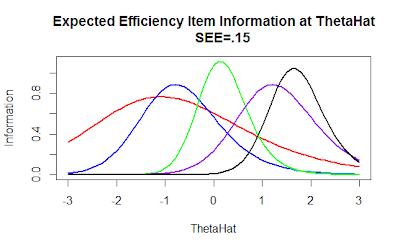## Friday, November 1, 2013

### Efficiency Balanced Information Criterion for Item Selection

```# Han (2012) in the paper "An Efficiency Balanced Information Criterion
# for Item Selection in Computerized Adaptive Testing" proposes a method
# of evaluating potential items based on expected item potential information
# as a function of maximum potential item information.

# This method favors items which have lower a values to be initially
# selected when there is greater uncertainty in the test but favors selection
# of items with higher a parameters as the test progresses.

# This small bit of code demonstrates how such a proceedure rescales
# item information.

# First we will define a few functions that we will use to construct our scale.

# Birbaum approximates the theta which maximizes the information function at
# a specific a, b, and c parameter level:
tmax <- function(a,b,c,D=1.7)
b+1/(D*a)+log((1+sqrt(1+8*c))/2)

# For example:
tmax(a=2,b=2,c=.2)

# This is the item information function for a 3PL (3 parameter logistic)
iinfo <- function(theta,a,b,c,D=1.7)
((D*a)^2*(1-c))/((c+exp(D*a*(theta-b)))*
(1+exp(-D*a*(theta-b)))^2)

iinfo(theta=0,a=1,b=0,c=.1)

# Now we define a function which approximates the integration of function
# "fun" from start to end.
integ <- function(start,end, step, fun, ...) {
x <- seq(start+step/2,end-step/2,step)
sum(get(fun)(x, ...)*step)
}
# As step size goes to zero the integ function approaches true integration.
# Of course that would mean infinite calculations which would be impossible
# for any computer.  Thus a larger step size is a worse approximation but
# uses less machine time.

# For example
a <- function(x,y) x^y

# Let's see
integ(0,2,.00001, "a", y=0)
integ(0,2,.00001, "a", y=1)
# Looking good.

# This is the big function that we are interested in:
IE <- function(thetahat,SEE,a,b,c,D=1.7,step=.001) {
# thetahat is the current estimate of ability
# SSE is the current standard error of the estimate
# step is the number of steps used to estimate the integral

# We calculate the item information at the current thetahat
ii <- iinfo(thetahat,a=a,b=b,c=c,D=D)
# Now we calculate the "max" theta value for the item.
thetamax <- tmax(a=a,b=b,c=c,D=D)
# Now the max information for that item.
maxI <- iinfo(thetamax,a=a,b=b,c=c,D=D)
# The efficient information as defined by Han at the
# current theta is:
ie <- ii/maxI

# einfo is the expected information for a particular
# item integrated across the range thetahat-SEE to
# thetahat+SEE.
einfo <- integ(thetahat-SEE*2,
thetahat+SEE*2,
step=step,
"iinfo",
a=a,b=b,c=c,D=D)

# Finally we can rescale the expected item information
# by the maxI to find the expected item efficiency.
eie <- einfo/maxI

# This provides a list of returned values.
list(eie=eie,
ii=ii,
ie=ie,
maxI=maxI,
thetamax=thetamax,
einfo=einfo)
}

test <- IE(0,1,a=1,b=0,c=.1,step=.001)
test

# Let's see this criterion in action:
theta <- seq(-3,3,.1)

# Make a list of returns
returns <- names(test)

for(v in returns) assign(v,NULL)

# Let's create one last function that returns a list of
# mappings for each of the ability levels.

mapping <- function(theta=seq(-3,3,.1), SEE=.5,a=1,b=0,c=.1,step=.001) {
I1 <- list()
for(i in 1:length(theta)) {
res <- IE(theta=theta[i],SEE=SEE,a=a,b=b,c=c,step=step)
for(v in returns) I1[[v]][i] <- res[[v]]
}
I1
}

# Now let's imagine five different items
I1 <- mapping(a=.5 , b=-1.5, c=.3, SEE=.5)
I2 <- mapping(a=1  , b=-1  , c=.3, SEE=.5)
I3 <- mapping(a=1.7, b=0   , c=.3, SEE=.5)
I4 <- mapping(a=1  , b=1   , c=.3, SEE=.5)
I5 <- mapping(a=1.5, b=1.5 , c=.3, SEE=.5)

plot(theta , I3\$ii, type="n",
main="Item Information at ThetaHat
SEE=.5",
xlab="ThetaHat", ylab="Information")
lines(theta, I1\$ii, lwd=2, col="red")
lines(theta, I2\$ii, lwd=2, col="blue")
lines(theta, I3\$ii, lwd=2, col="green")
lines(theta, I4\$ii, lwd=2, col="purple")
lines(theta, I5\$ii, lwd=2, col="black")
# than other items such that they would almost never
# be selected.  Item 4 for instance is almost never expected
# to yeild higher information.# If we are less sure of our theta estimate we may instead
# calculate our expected information.
plot(theta , I3\$einfo, type="n",
main="Expected Item Information at ThetaHat
SEE=.5",
xlab="ThetaHat", ylab="Information")
lines(theta, I1\$einfo, lwd=2, col="red")
lines(theta, I2\$einfo, lwd=2, col="blue")
lines(theta, I3\$einfo, lwd=2, col="green")
lines(theta, I4\$einfo, lwd=2, col="purple")
lines(theta, I5\$einfo, lwd=2, col="black")
# In general this basically makes the peaks less extreme but
# does not generally favor our items with lower a values. ```
``````
```# If we want to see how our expected efficiency item
# information value will do we can see that as well.
# However, before we do that imagine first each of these
# information functions divided by it's peak value.
plot(c(0,theta) , c(0,I1\$eie), type="n",
main="Expected Efficiency Item Information at ThetaHat
SEE=.5",
xlab="ThetaHat", ylab="Information")
lines(theta, I1\$eie, lwd=2, col="red")
lines(theta, I2\$eie, lwd=2, col="blue")
lines(theta, I3\$eie, lwd=2, col="green")
lines(theta, I4\$eie, lwd=2, col="purple")
lines(theta, I5\$eie, lwd=2, col="black")
# Now we can see that item 1 (red) and 4 (purple) are favored by
# this algorithm, though by standard item maximization or by
# expected item maximization they would almost never have been
# chosen. ```
```# The authors suggest a summing or the Efficiency Information
# and that of expected information might yeild a good solution.
plot(c(0,theta) , c(0,I3\$eie+I3\$einfo), type="n",
main="Expected Efficiency Item Information at ThetaHat
SEE=.5",
xlab="ThetaHat", ylab="Information")
lines(theta, I1\$eie+I1\$einfo, lwd=2, col="red")
lines(theta, I2\$eie+I2\$einfo, lwd=2, col="blue")
lines(theta, I3\$eie+I3\$einfo, lwd=2, col="green")
lines(theta, I4\$eie+I4\$einfo, lwd=2, col="purple")
lines(theta, I5\$eie+I5\$einfo, lwd=2, col="black")# The argument is that as SEE gets small the information begins
# to look much more like that of Item Information which is
# appropropriate for later in the test.
I1 <- mapping(a=.5 , b=-1.5, c=.3, SEE=.15)
I2 <- mapping(a=1  , b=-1  , c=.3, SEE=.15)
I3 <- mapping(a=1.7, b=0   , c=.3, SEE=.15)
I4 <- mapping(a=1  , b=1   , c=.3, SEE=.15)
I5 <- mapping(a=1.5, b=1.5 , c=.3, SEE=.15)

plot(c(0,theta) , c(0,I3\$eie), type="n",
main="Expected Efficiency Item Information at ThetaHat
SEE=.15",
xlab="ThetaHat", ylab="Information")
lines(theta, I1\$eie, lwd=2, col="red")
lines(theta, I2\$eie, lwd=2, col="blue")
lines(theta, I3\$eie, lwd=2, col="green")
lines(theta, I4\$eie, lwd=2, col="purple")
lines(theta, I5\$eie, lwd=2, col="black")
# Now we can see that item 1 (red) and 4 (purple) are favored by
# this algorithm, though by standard item maximization or by
# expected item maximization they would almost never have been
# chosen.# The authors suggest a summing or the Efficiency Information
# and that of expected information might yeild a good solution.
plot(c(0,theta) , c(0,I3\$eie+I3\$einfo), type="n",
main="Expected Efficiency Item Information at ThetaHat
SEE=.15",
xlab="ThetaHat", ylab="Information")
lines(theta, I1\$eie+I1\$einfo, lwd=2, col="red")
lines(theta, I2\$eie+I2\$einfo, lwd=2, col="blue")
lines(theta, I3\$eie+I3\$einfo, lwd=2, col="green")
lines(theta, I4\$eie+I4\$einfo, lwd=2, col="purple")
lines(theta, I5\$eie+I5\$einfo, lwd=2, col="black")# We can see that item 1 is still favored though we expected
# it to give us very little information.  Overall, the
# method seems interesting but not yet ideal.```
Created by Pretty R at inside-R.org